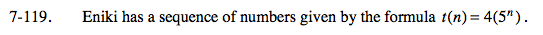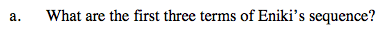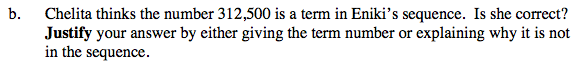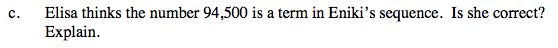Home > A2C > Chapter 7 > Lesson 7.2.2 > Problem7-119

7-119.
1. Eniki has a sequence of numbers given by the formula t(n) = 4(5n). Homework Help ✎

1. What are the first three terms of Eniki's sequence?

2. Chelita thinks the number 312,500 is a term in Eniki's sequence. Is she correct? Justify your answer by either giving the term number or explaining why it is not in the sequence.

3. Elisa thinks the number 94,500 is a term in Eniki's sequence. Is she correct? Explain.Substitute n = 1, 2, 3 into the formula.Let t(n) = 312,500 and solve for n. Be sure to divide both sides of the equation by 4 before taking the log of both sides.

What kinds of numbers will tell you if it is in the sequence?See part (b).